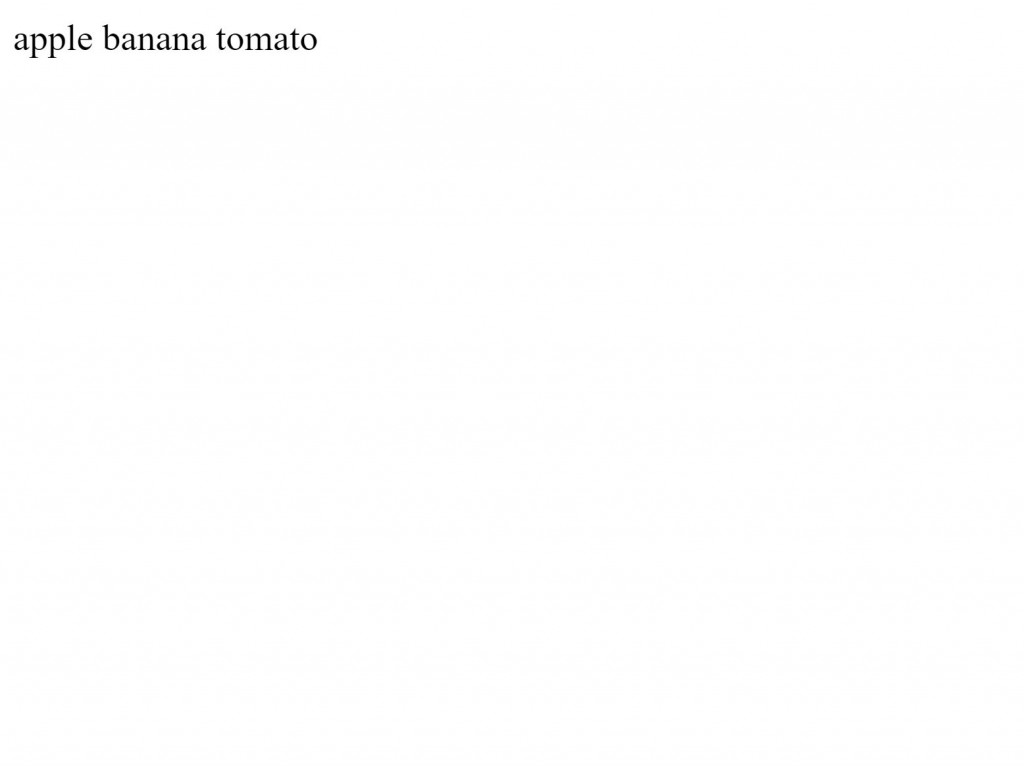#DAY 22
0
Modern Web

## 陣列

### 新建陣列

``````var a=new Array();
``````

``````var a=new Array();
a='John';
a='Mary';
a='Tom';
console.log(a) // 印出 ['John','Mary','Tom']
``````

### 索引 (index)

`a='Mary'` 就是在第二個位置將字串 Mary 放進去

`a='Tom'` 就是在第三個位置將字串 Tom 放進去

### 存取陣列中的元素

``````array[index]
``````

``````var a=new Array();
a='John';
a='Mary';
a='Tom';
console.log(a) // 印出 'John'
console.log(a) // 印出 'Mary'

``````

### 陣列實字

``````var a=[];  // 建立空陣列
a=1;    // 將 1 放進第一個資料
a=2;    // 將 2 放進第二個資料
a=3;
``````

``````var a=['apple','banana','Tomato']; // 建立陣列同時將資料指定進去。
``````

### 陣列長度

``````var a=['apple','banana','Tomato'];
console.log(a.length);  // 印出 3
``````

### 將資料自動放進網頁

``````var a=['apple','banana','tomato'];

for(var i=0;i<a.length;i++){
console.log(a[i]);
}
``````

``````<body>
<div id="root">
</div>
<script>
var a=['apple','banana','tomato'];
var root=document.getElementById('root');
for(let i=0;i<a.length;i++){
root.innerHTML+=a[i]+" ";
}
</script>
</body>
``````